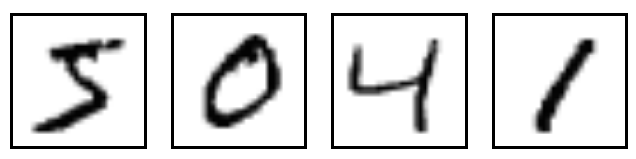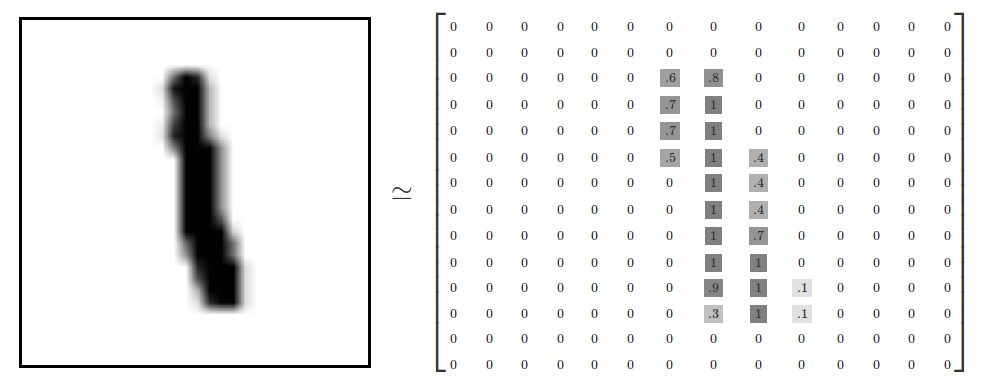The Convolutional Neural Networks or “ConvNets” gained popularity through their use with image data, and are currently the state of the art for identifying faces, objects and traffic signs apart from powering vision in robots and self driving cars. In this tutorial we’ll work with MNIST dataset. MNIST is a simple computer vision dataset. It consists of images of handwritten digits like the image below. We will then train a ConvNet model on the training set using TensorFlow and make predictions on a test set. Please read my previous post on implementation of a basic neural netwok on the same dataset.Resources:

Contents

The basic CNN structure is as follows: Convolution -> Pooling -> Convolution -> Pooling -> Fully Connected Layer -> OutputThere are three main operations in the ConvNet shown in Figure 3 above:

1. Convolution: Convolution is the act of taking the original data, and creating feature maps from it. The convolutional layers are not fully connected like a traditional neural network.
2. Pooling or Sub Sampling: Pooling is sub-sampling, most often in the form of “max-pooling,” where we select a region, and then take the maximum value in that region, and that becomes the new value for the entire region.
3. Classification (Fully Connected Layer): Fully Connected Layers are typical neural networks, where all nodes are “fully connected.”

These operations are the basic building blocks of every Convolutional Neural Network, so understanding how these work is an important step to developing a sound understanding of ConvNets.

## Understanding and Importing the MNIST Data

We’re going to be working first with the MNIST dataset, which is a dataset that contains 60,000 training samples and 10,000 testing samples of hand-written and labeled digits, 0 through 9, so ten total “classes.”

The MNIST dataset has the images (see example above), which we’ll be working with as purely black and white, thresholded, images, of size 28 x 28, or 784 pixels total.Our features will be the pixel values for each pixel, thresholded. Either the pixel is “blank” (nothing there, a 0), or there is something there (1). Those are our features. We’re going to attempt to just use this extremely rudimentary data, and predict the number we’re looking at (a 0,1,2,3,4,5,6,7,8, or 9).

``````import tensorflow as tf

from tensorflow.examples.tutorials.mnist import input_data
mnist = input_data.read_data_sets("/tmp/data/", one_hot = True)
``````
``````Extracting /tmp/data/train-images-idx3-ubyte.gz
Extracting /tmp/data/train-labels-idx1-ubyte.gz
Extracting /tmp/data/t10k-images-idx3-ubyte.gz
Extracting /tmp/data/t10k-labels-idx1-ubyte.gz
``````

The MNIST data is split into three parts: 55,000 data points of training data (mnist.train), 10,000 points of test data (mnist.test), and 5,000 points of validation data (mnist.validation).

For the purposes of this tutorial, we’re going to want our labels as “one-hot vectors”. A one-hot vector is a vector which is 0 in most dimensions, and 1 in a single dimension. In this case, the nth digit will be represented as a vector which is 1 in the nth dimension. For example, 3 would be [0,0,0,1,0,0,0,0,0,0]. Consequently, mnist.train.labels is a [55000, 10] array of floats.

## Setting up the Computation Model

### Defining Variables

To begin, we’re mostly simply defining some starting variables, the class-size, and then we’re defining the batch size to be 128.

``````# import rnn from tensorFlow

batch_size = 128

# define number of classes = 10 for digits 0 through 9
n_classes = 10

# tf Graph input
x = tf.placeholder(tf.float32, [None, 784])
y = tf.placeholder(tf.float32, [None, n_classes])
``````

### Functions for Convolution and Pooling

Next, we’re going to define a couple simple functions that will help us with our convolutions and pooling. The functions here are the exact same as the ones from the offical TensorFlow CNN tutorial.

• The strides parameter dictates the movement of the window. In this case, we just move 1 pixel at a time for the conv2d function.
• The ksize parameter is the size of the pooling window and we move 2 pixel at a time.
• Padding refers to operations on the windows at the edges, it is like a reflective boundary condition.
``````def conv2d(x, W):
return tf.nn.conv2d(x, W, strides=[1, 1, 1, 1], padding='SAME')

def maxpool2d(x):
return tf.nn.max_pool(x, ksize=[1, 2, 2, 1], strides=[1, 2, 2, 1], padding='SAME')
``````

### Convolutional Neural Network model

We have a weights/biases dictionary like basic deep nets, but they depend on specific convolutions algorithm as follows:

** Definitions of the layers **

• Each input image had a 28x28 pixels
• We’re taking 5x5 convolutions on the initial image, and producing 32 outputs. (Not set in stone, pick any similar number)
• Next, we take 5x5 convolutions of the 32 inputs and make 64 outputs.
• From here, we’re left with 64 of 7x7 sized images (It went through two max pooling process. And each time, it reduces the dimension by half because of its stride of 2 and size of 2. Hence, 28/2/2 = 7)
• then we’re outputting to 1024 nodes in the fully connected layer.
• Then, the output layer is 1024 layers, to 10, which are the final 10 possible outputs for the actual label itself (0-9).

** Apply appropriate functions to the layers **

• Reshape input to a 4D tensor
• Apply Non Linearity (ReLU) on the convoluted output (conv1)
• The ReLU rectifier is an activation function defined as f(x)=max(0,x)
• Apply pooling and repeat the previous two steps for layer 2 (conv2)
• Reshape conv2 output to fit fully connected layer
• Apply ReLU on the fully connected layer
• Obtain the output layer
``````def convolutional_neural_network(x):#, keep_rate):
weights = {
# 5 x 5 convolution, 1 input image, 32 outputs
'W_conv1': tf.Variable(tf.random_normal([5, 5, 1, 32])),

# 5x5 conv, 32 inputs, 64 outputs
'W_conv2': tf.Variable(tf.random_normal([5, 5, 32, 64])),

# fully connected, 7*7*64 inputs, 1024 outputs
'W_fc': tf.Variable(tf.random_normal([7*7*64, 1024])),

# 1024 inputs, 10 outputs (class prediction)
'out': tf.Variable(tf.random_normal([1024, n_classes]))
}

biases = {
# Biases only depend on the outputs of a layer from above
'b_conv1': tf.Variable(tf.random_normal()),
'b_conv2': tf.Variable(tf.random_normal()),
'b_fc': tf.Variable(tf.random_normal()),
'out': tf.Variable(tf.random_normal([n_classes]))
}

# Reshape input to a 4D tensor
x = tf.reshape(x, shape=[-1, 28, 28, 1])

# Convolution Layer, using our function and pass it through ReLU
conv1 = tf.nn.relu(conv2d(x, weights['W_conv1']) + biases['b_conv1'])

# Max Pooling (down-sampling)
conv1 = maxpool2d(conv1)

# Convolution Layer
conv2 = tf.nn.relu(conv2d(conv1, weights['W_conv2']) + biases['b_conv2'])

# Max Pooling (down-sampling)
conv2 = maxpool2d(conv2)

# Fully connected layer
# Reshape conv2 output to fit fully connected layer
fc = tf.reshape(conv2, [-1, 7*7*64])
fc = tf.nn.relu(tf.matmul(fc, weights['W_fc']) + biases['b_fc'])

# Output Layer
output = tf.matmul(fc, weights['out']) + biases['out']
return output
``````

## Training the Model

Under a new function, train_neural_network, we will pass our output data.

• We then produce a prediction based on the output of that data through our convolutional_neural_network().
• Next, we create a cost variable. This measures how wrong we are, and is the variable we desire to minimize by manipulating our weights. The cost function is synonymous with a loss function.
• To optimize our cost, we will use the AdamOptimizer, which is a popular optimizer along with others like Stochastic Gradient Descent and AdaGrad, for example.
• Within AdamOptimizer(), we can optionally specify the learning_rate as a parameter. The default is 0.001, which is fine for most circumstances.
• Now that we have these things defined, we begin the session.
``````hm_epochs = 4

def train_neural_network(x):
prediction = convolutional_neural_network(x)
cost = tf.reduce_mean( tf.nn.softmax_cross_entropy_with_logits(logits=prediction,labels=y) )

hm_epochs = 10
with tf.Session() as sess:
sess.run(tf.global_variables_initializer())

for epoch in range(hm_epochs):
epoch_loss = 0
for _ in range(int(mnist.train.num_examples/batch_size)):
epoch_x, epoch_y = mnist.train.next_batch(batch_size)
_, c = sess.run([optimizer, cost], feed_dict={x: epoch_x, y: epoch_y})
epoch_loss += c

print('Epoch', epoch, 'completed out of',hm_epochs,'loss:',epoch_loss)

correct = tf.equal(tf.argmax(prediction, 1), tf.argmax(y, 1))

accuracy = tf.reduce_mean(tf.cast(correct, 'float'))
print('Accuracy:',accuracy.eval({x:mnist.test.images, y:mnist.test.labels}))

train_neural_network(x)
``````
``````Epoch 0 completed out of 10 loss: 1237213.87835
Epoch 1 completed out of 10 loss: 249016.677132
Epoch 2 completed out of 10 loss: 145539.957864
Epoch 3 completed out of 10 loss: 95145.4681053
Epoch 4 completed out of 10 loss: 66667.0109224
Epoch 5 completed out of 10 loss: 52665.541432
Epoch 6 completed out of 10 loss: 44668.1030514
Epoch 7 completed out of 10 loss: 29775.3795409
Epoch 8 completed out of 10 loss: 24104.3042541
Epoch 9 completed out of 10 loss: 20951.965727
Accuracy: 0.9792
``````

## Accuracy and Conclusion

Our basic neural network model gave an accuracy of ~95% for 10 epochs. This ConvNet model certainly gives better accuracy of ~98% for the same number of epochs.